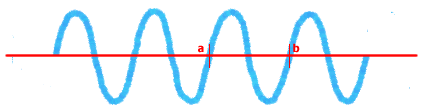#### Reference : Frequency Bands

Frequency describes how many wave cycles there are in a period of 1 (one) second. Frequency of a wave is inverse proportional with its Period. Frequency is denoted in cycle per second or Hertz (Hz).

 f = 1/T where f is frequency in Hertz and T is period in second.Figure: Wave. The time it takes for the wave to travel from point "a" to point "b" is called a Period (T).

Frequency discussion has always been an integral part of any telecommunication subject be it wired or wireless because telecommunication is about sending and receiving electromagnetic (EM) waves.

Another parameter of an EM wave is wavelength. The relation between frequency and wavelength of an EM wave is illustrated in this equation:

 c = λf where c is the speed of light (= 3x108 m/s), λ is wavelength in meter, f is frequency in Hertz.

Frequency band designation is given by standard bodies and the telecom industry based on characteristic of each frequency range. The following tables list frequency bands that are publicly known and often mentioned in telecommunication literature.

 Back Next